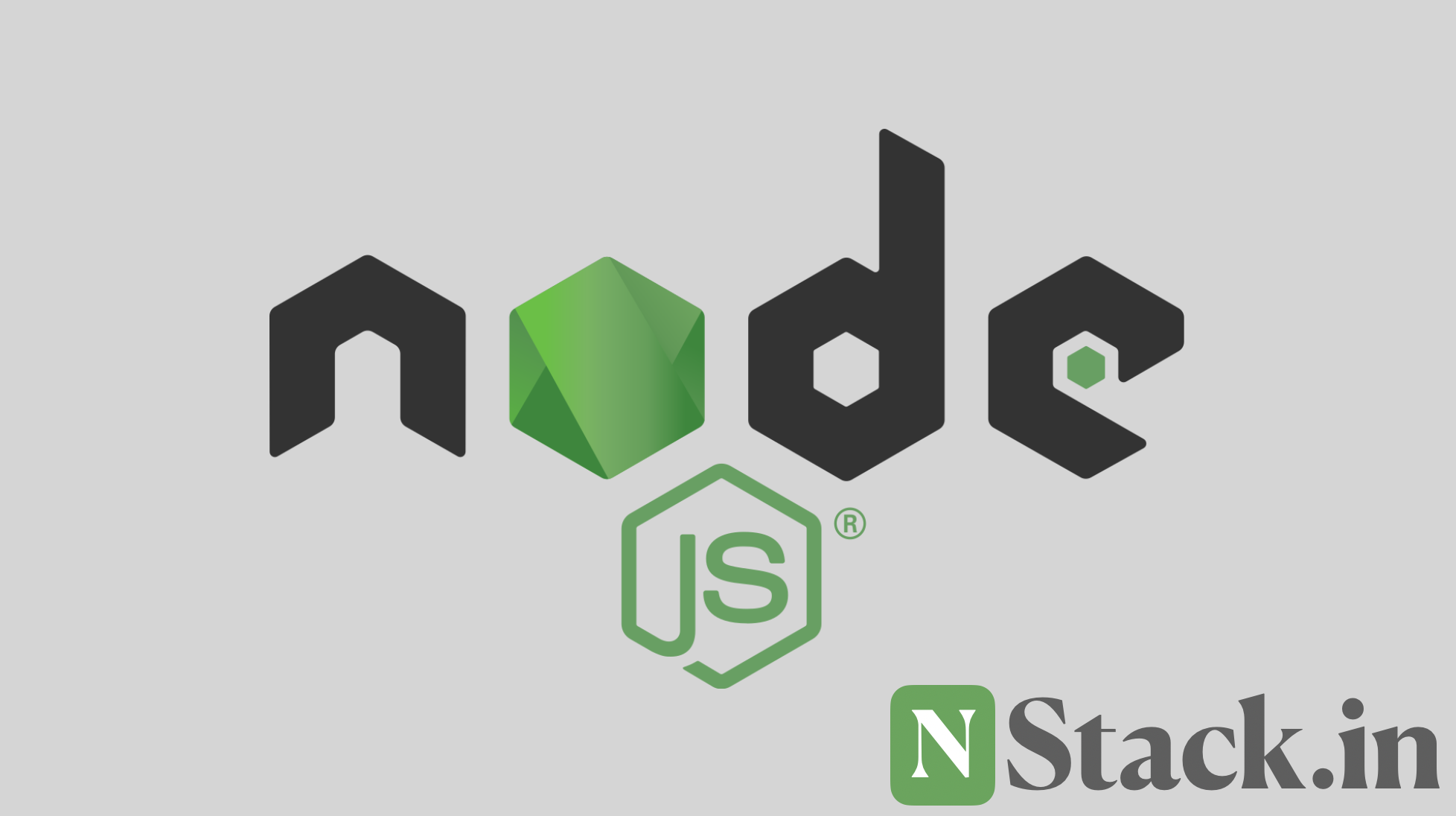## Variables in Node.js #

In programming language there is variables which is used to store data and used to refer that data. This is one of the important things which any programer should know.

You can store any kind of data in variables and used it further in the program. Let’s see the how to use the variable.

• Define Variable
• Store Data in Variable

## Define Variable #

There are some rules which is must know to know about variables.

• Variables declaration starts with `var`
• Variables can contain alphabet, numbers, \$ (dollar) and _ (underscore).

#### valid variables #

Let’s see some of the valid variable names.valid-variable.js
``````var num; // Number can contain alphabet
var \$number; // Number can contain alphabet
var number1; // Number can be used with variable name
var number_one; // underscore can be used in variable
``````

#### in-valid variables #

Let’s see some of the in-valid variable names.in-valid-variable.js
``````var 12num;            // Variable can't start with number
var number 1;         // Variable can't contain space
var \number_one;      // variable can't contain special character other than _ and \$
``````

You see how you